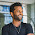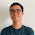### leetcode Question 132: Palindrome Partitioning

Palindrome Partitioning

Given a string s, partition s such that every substring of the partition is a palindrome.
Return all possible palindrome partitioning of s.
For example, given s = "aab",
Return
  [
["aa","b"],
["a","a","b"]
]

Analysis:
When face the "return all", "get all ", "find all possible", "find the total number of", an idea is to use the recursion. Same as this problem!

To get the all the partitions of a string s:
1. find all the palindromes in substring s, and all the palindromes in substring s[1:end]
2. find all the palindromes in substring s[0:1], and all the palindromes in substring s[2:end]
...
find all the palindromes in substring s[1:end-1], and all the palindromes in substring s[end]

So the problem is quite clear, when we do recursion, two things should be considered:
1. stop condition:  when the search goes to the last position in the string
2. for loop or while loop:   for position=current start position to the end.

This problem is not complex, see the code below and you will understand the idea:

Code:
class Solution {
public:

bool valid(string &str, int st, int ed){
while (st<ed){
if (str[ed]!=str[st]){
return false;
}else{
st++;
ed--;
}
}
return true;
}

void find(string s, int st, vector<string> &r, vector<vector<string> > &res){
if (st>=s.size()){
res.push_back(r);
}else{
for (int i=st;i<s.size();i++){
if (valid(s,st,i)){
r.push_back(s.substr(st,i-st+1));
find(s,i+1,r,res);
r.pop_back();
}

}
}
}

vector<vector<string>> partition(string s) {
// Start typing your C/C++ solution below
// DO NOT write int main() function
vector<vector<string> > res;
vector<string> r;
find(s,0,r,res);
return res;
}
};


1.One question: find() will be invoked duplicately for a given string range s[i..n], right? So why don't use some data structure to save the intermediate results, that is, using the DP idea?

1.@Qiang I didn't find any duplicate node in the recursion tree so that I could apply DP. I think the tree has no duplicate node. Can you give me any testcase?

2.What is the complexity of this soln?

3.whats the time complexity ?

4.This is my discussion and Java implementation http://www.capacode.com/dynamic-programming/split-string-into-palindromes/. You can also find testcases to test your program.